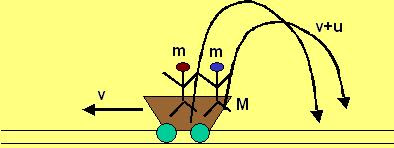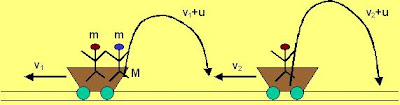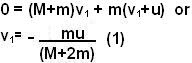## Monday, May 12, 2008

### Irodov Problem 1.156a) The total momentum of the (buggy + two men) system will be conserved just before and after their jump. Initially everyone is at rest and so the total momentum is 0. Suppose that after the men jump the buggy moves with a velocity v, then its momentum will be Mv. Since the men jump into the air with a speed u relative to the buggy, their speed in air with respect to a stationary observer will be v+u, and so their combined momentum in air will be 2m(v+u). Hence we have,(b) We have to solve this problem in two parts, first after the first man jumps and then after the second man jumps. Suppose that after the first man jumps, the buggy attains a velocity v1 and then suppose that after the second man jumps it attains a velocity v2.

Just before the first man jumps off the system is at rest and so the total momentum is 0. Just after the first man jumps, the momentum of buggy and one remaining person will be (M+m)v1. The man jumps with a speed u relative to the buggy and so his speed in the air relative to a stationary reference will be m(v1+u). Since, there are no external forces, the momentum of the (buggy + 2 men) system just before and after the first man jumps will be conserved. So we have,Now the second man jumps. Before he jumps the momentum of the (buggy + remaining man) is given by (M+m)v1. After the man jumps, the momentum of the buggy becomes Mv2. Since the man jumps with a speed u relative to the buggy his speed in air relative to a stationary observer is v2+u and his momentum in air m(v2+u). Since there are no external forces on the (remaining man + buggy) system before and after his jump, the momentum of the (remaining man + buggy) system must be conserved. So we have,